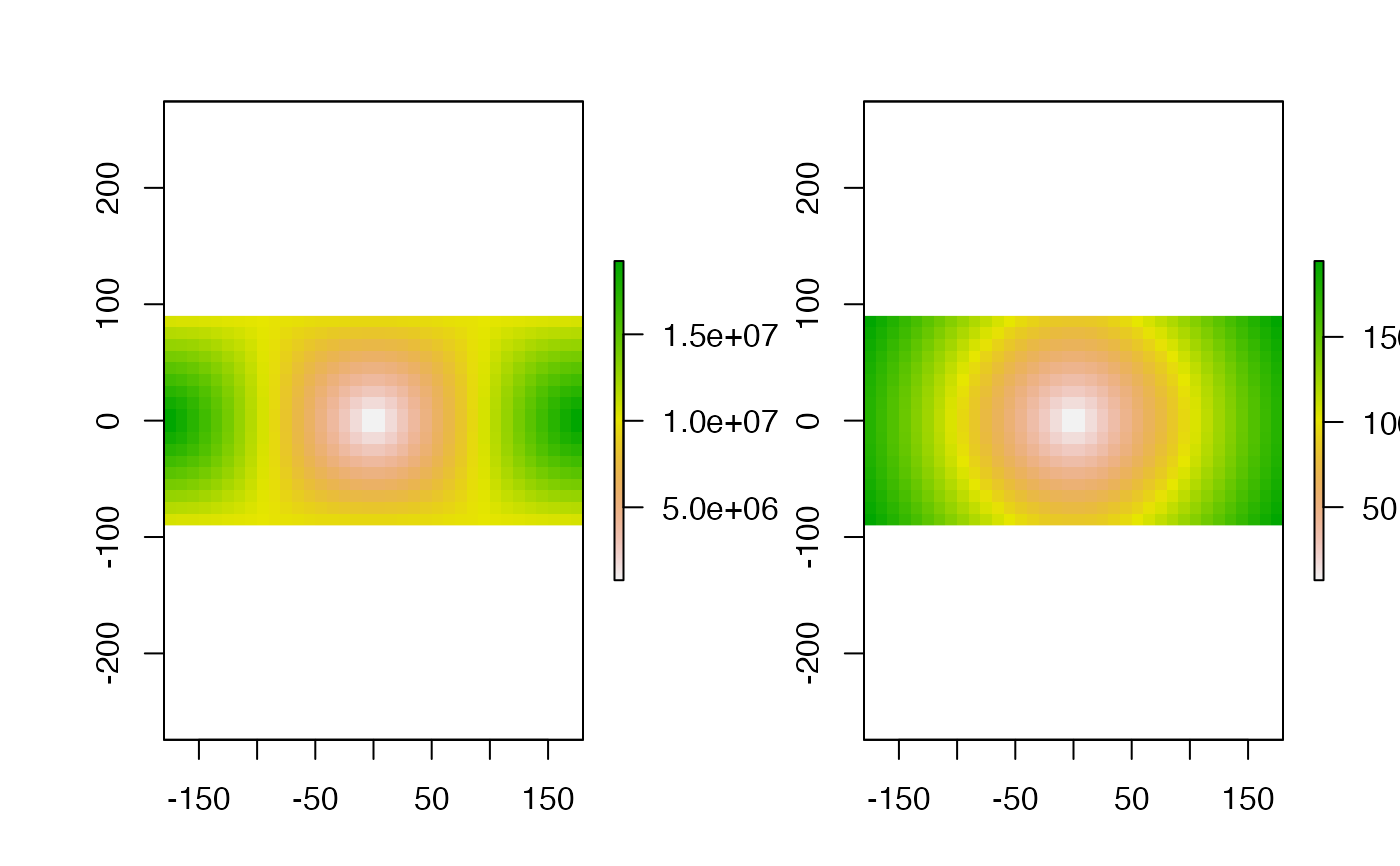The function calculates the distance from a set of points to all cells of a Raster* object.

The distance unit is in meters if the coordinate reference system (crs) of the Raster* object is (+proj=longlat) or assumed to be if the crs is NA. In all other cases it is in the units defined by the crs (which typically is meters).

distanceFromPoints(object, xy, filename='', ...)

## Arguments

object

Raster object

xy

matrix of x and y coordinates, or a SpatialPoints* object.

filename

character. Optional filename for the output RasterLayer

...

Additional arguments as for writeRaster

## Details

Distances for longlat data are computed on the WGS84 spheroid using GeographicLib (Karney, 2013)

## Value

RasterLayer

crs, distance, gridDistance, pointDistance

## Examples

r <- raster(ncol=36,nrow=18)
xy <- c(0,0)
d1 <- distanceFromPoints(r, xy)
crs(r) = '+proj=utm +zone=12 +datum=WGS84'
d2 <- distanceFromPoints(r, xy)
par(mfrow=c(1,2))
plot(d1)
plot(d2)